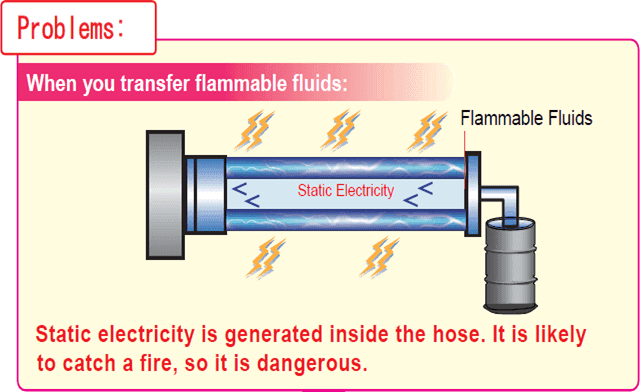Resin Hose Manufacturer in Japan

# Hose/Tube for Flammable Fluids

• HOME »
• Hose/Tube for Flammable FluidsWhen transporting flammable liquids (combustible fluid), dealing with static electricity is necessary in all fields. (For instance, in the field of electronics, the compliance to ATEX requirements is absolutely necessary.) Hydrocarbon fluids (used in fuel oil and cleaning agents) are highly insulating and static fluids. In the dry winter, the measurement against the static electricity is absolutely required. For the transport of highly flammable liquids, we recommend you to use “Flexible Fluorine (ETFE) Resin Hose Series (Dissipative Type).” “Flexible Fluorine (ETFE) Resin Hose Series (Dissipative Type)” is the best solution for the streaming electrification generated inside the hose. (Flexible Fluorine (ETFE) Resin Hose Series (Dissipative Type) performs better than a hose with ground wire, in terms of non-charging static electricity.)

In order to understand “Flexible Fluorine (ETFE) Resin Hose Series (Dissipative Type)” more easily, please take a look at the short video clip of “Flexible Fluorine (ETFE) Resin Hose Series (Dissipative Type).”

## Case Studies

A: Printing and Paint Customer

Fluids: Inks and Paints
Current Problems: Conductive rubber hose does not any chemical resistance. Internal cost goes up as the frequency of changing hose goes up.
Solutions: HAKKO recommends “E-SJSD: Flexible Fluorine (ETFE) Resin SUS Spring Wire Hose (Dissipative Type).” This is because E-SJSD has the features of conductivity and solvent resistance. As a result of these features, the frequency of changing a hose is far less often.

Fluids: Toluene (Organic Solvents)
Current Problems: This customer is currently using a teflon hose, but no measurements against the generation of static electricity are taken. It is possible to catch a fire.
Solutions: HAKKO recommends “E-SJSD: Flexible Fluorine (ETFE) Resin SUS Spring Wire Hose (Dissipative Type).” This is because E-SJSD has the features of conductivity and solvent resistance. E-SJSD solves the problem of the generation of static electricity.

C: Fragrance and Aromatic Customer

Fluids: Gel
Current Problems: PVC hoses have been used, but the local authority demands that a hose has to have measurements against the generation of static electricity.
Solutions: HAKKO recommends “E-SJSD: Flexible Fluorine (ETFE) Resin SUS Spring Wire Hose (Dissipative Type).” This is because E-SJSD has the features of conductivity and solvent resistance. E-SJSD meets the inspection requirements of the local authority.

D: Powder Customer

Fluids: Iron Powder
Current Problems: PVC hoses have been used, but the static electricity was generated after the friction of iron powder.
Solutions: HAKKO recommends “E-SJD: Flexible Fluorine (ETFE) Resin Tubing (Dissipative Type).” This is because E-SJD has the feature of conductivity. E-SJD solves the problem of static electricity.

### Frequently Asked Question on Why E-SJD/E-SJSD are suitable for Flammable Fluids

• In the technical specifications, issued by the International Electrotechnical Commission (IEC) as the “Safety Guide for Hoses,” Guidelines for static electricity have been defined. (IEC/TS 60079 -32 -1: 2013 7.7.3 Hose and hose assembly). To minimize the danger from the static electricity and the stray current, the regulation on the classification of resistance exists between one end of the hose and the other end of the hose.
• These are used as the hose end-to-end resistance (R) standard, which is shown below:
• “Conductivity (R < 1 KΩ)”; “Dissipative (1 KΩ < R < 1 MΩ)”; “Insulation (1MΩ ≦ R)”
• Among these standards above, in the case of conductive (R < 1 KΩ) piping, it is easy to be affected by stray current and difficult to control at a safe level. Stray current leads to the situation that the current leaks from the electrical equipment to the ground. We think that this leakage is not acceptable. We believe that the “dissipative” level is the most appropriate. Thus, we recommend HAKKO E-SJD/SJSD, dissipative product series.

## Electrical Properties of Major Flammable Fluids (Conductivity)

The following information is cited from “Industrial Safety and Health Research Institute Technical Guidance on Electrostatic Safety Guide 2007 ISSN 1882 -2703 JNIOSH-TR-NO. 42 (2007) page 157 – 162 G.5: Electrical Properties of Fluids.”

#### Insulating Fluid (<100 pS/m)

Fluid
Conductivity pS/m
Fluid
Conductivity pS/m
Anisole (Methylphenyl Ether)10Turpentine22
Ethylbenzene30Diesel Oil Light Oil （Purified）About 0.1
Caprylic Acid (Octanoic Acid)<37Toluene<1
Xylene0.1Carbon Disulfide (1$^\circ$C) (34$^\circ$F)7.8$\times$10-4
Cyclohexane<2Hexamethyldisilazane29
Diethyl Ether30Hexane1$\times$10-5
Jet Fuel（A,A-1,B）0.01 - 50Heptane3$\times$10-2
1,4-Dioxane (Diethylenedioxite)0.1Benzene5$\times$10-3
Styrene Monomer10

#### Semi-Conductive Fluid (100 – 10,000 pS/m)

Fluid
Conductivity pS/m
Fluid
Conductivity pS/m
2-Ethylhexyl Acrylate610Pentyl 1-Acetate (- Amyl.) (23$^\circ$C) (73$^\circ$F)2160
Butyl Acrylate (Butyl Acrylate)3580n-Butyl Acetate (23$^\circ$C) (73$^\circ$F)4700
Isopropyl Ether500n-Propyl Acetate (4$^\circ$C) (39$^\circ$F)8460
2-Ethylhexyl Alcohol79001,2,4-Trichlorobenzene200
Chlorobenzene7000Propionic Acid (25$^\circ$C) (77$^\circ$F)8500
Dichloroethylene40001-Bromonaphthalene3660
Isobutyl Acetate (23$^\circ$C) (73$^\circ$F)4320Bromobenzene1200

#### Conductive Fluid (>10,000 pS/m)

Fluid
Conductivity pS/m
Fluid
Conductivity pS/m
Ethyl Acrylate3.35$\times$105Ethyl Acetate (23$^\circ$C) (73$^\circ$F)4.6$\times$104
Acrylonitrile7$\times$105Vinyl Acetate (23$^\circ$C) (73$^\circ$F)2.6$\times$104
Acetaldehyde (15$^\circ$C) (59$^\circ$F) 1.7$\times$108Diethanolamine (20$^\circ$C) (68$^\circ$F)4.6$\times$107
Acetone (25$^\circ$C) (77$^\circ$F)6$\times$106Diethylketone5.2$\times$107
Aniline2.4$\times$106Diethylerin Glycol5.86$\times$107
Allyl Alcohol7$\times$108Cyclohexanone5$\times$105
Isoamyl Alcohol1.4$\times$105Dichloroethane2.0$\times$105
Isopropyl alcohol (25$^\circ$C) (77$^\circ$F)3.5$\times$108Dimethylformamide6$\times$106
Ethanolamine1.1$\times$109Tetrahydrofuran3.3$\times$105
Ethylamine (0$^\circ$C) (32$^\circ$F)4$\times$107Triethylene Glycol8.4$\times$106
Ethyl Alcohol (25$^\circ$C) (77$^\circ$F)1.35$\times$105Nitrobenzene (0$^\circ$C) (32$^\circ$F)5$\times$105
Ethyleneimine8$\times$108Pyridine (18$^\circ$C) (64$^\circ$F)5.3$\times$106
Ethylene Glycol1.16$\times$108n-Butyl Alcohol (25$^\circ$C) (77$^\circ$F)2.0$\times$107
Ethylenediamine9$\times$106Furfural (25$^\circ$C) (77$^\circ$F)1.5$\times$108
Epichlorohydrin (25$^\circ$C) (77$^\circ$F)3.4$\times$106n-Propyl Alcohol (25$^\circ$C) (77$^\circ$F)2$\times$106
Formic Acid (25$^\circ$C) (77$^\circ$F)6.4$\times$109Benzyl Alcohol (25$^\circ$C) (77$^\circ$F)1.8$\times$108
Ethyl Formate1.45$\times$105n-Methyl -2 pyrrolidone2$\times$106
Methyl Formate1.92$\times$108Methyl Alcohol (18$^\circ$C) (64$^\circ$F)4.4$\times$107
Quinoline2.2$\times$106Methylisobutyl Ketone<5.2$\times$106
Glycerin (25$^\circ$C) (77$^\circ$F)6.4$\times$106Methyl ethyl ketone (25$^\circ$C) (77$^\circ$F)1$\times$107
M-cresol1.397$\times$106Methylformamide8$\times$107
Chlorohydrin (25$^\circ$C) (77$^\circ$F)5$\times$107Monoethanolamine (MEA/20$^\circ$C)1.2$\times$109
Acetic Acid (25$^\circ$C) (77$^\circ$F)1.12$\times$106-

## Major Product List

#### Flexible Fluorine (ETFE) Resin Tubing (Dissipative Type) [Model Number: E-SJD]

Conductive
Solvent Resistance
Non-PVC
Chemical Resistance
Low Odor
Food-Sanitation
UV Cut
Oil-Proof
Alcohol Resistance
UL Compliant

Inner Diameter : 6 - 8mm

#### Flexible Fluorine (ETFE) Resin SUS Spring Wire Hose (Dissipative Type) [Model Number: E-SJSD]

Conductive
Solvent Resistance
Non-PVC
Chemical Resistance
Low Odor
Food-Sanitation
UV Cut
Vacuum
Oil-Proof
Hard to Break
Alcohol Resistance
UL Compliant

Inner Diameter : 12 - 25mm

*If you are interested in transparent fluorine tubing with some measurements against the static electricity, please visit this webpage (SJ-AST: Flexible Fluorine (ETFE) Resin Tubing (Anti-Static Type)).